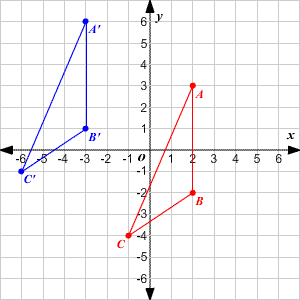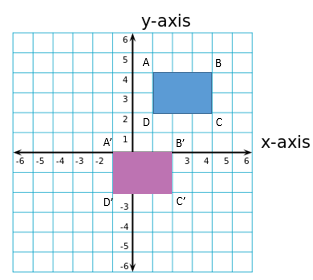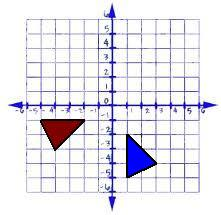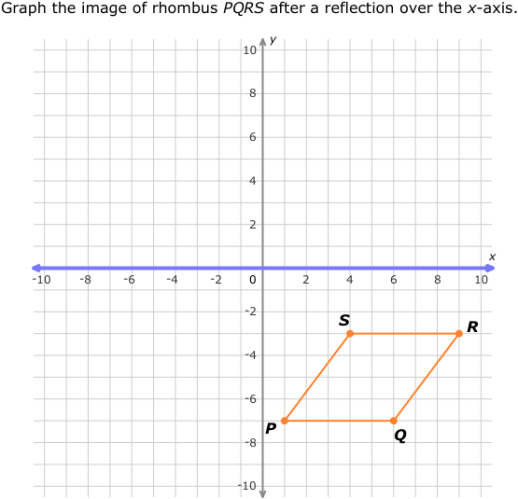Rotations
Reflections
Translations
All Transformations
3 Trivia & 2 jokes
100

All figures in math are always rotated in this direction, unless told otherwise.

What is counterclockwise?

100

The line of reflectionWhat is the x-axis?

100

Write a rule to describe the translation below.What is x, y > (x - 5), (y + 3)?

Or  What is left 5, up 3?

100

Rotation is a change in the figure by _________ it.

What is turning it?

100

Trivia: The number of spectators allowed at indoor games at New Bern High School.

What is 25?

200

The rule for rotating a figure 90 degrees

What is  x, y > (-y, x)  which means change the y and then switch the x and the y to draw the image?

200

The line of reflections?What is the y-axis?

200

Write a rule to describe the translation.What is x, y> (x-2, y-4)?

or

What is left 2, down 4?

200

The name for the point (0, 0).

What is the origin?

200

Trivia:   The spelling for Ms Miller's middle name.

What is L-o-r-r-a-i-n-e?

300

The coordinates for F' after a 90 degree rotation.What is (-5, 2).

Explanation: Change y from 2 to -2, then switch the x and the y.  (2, 5) becomes (-5, 2)

300

The coordinates of the pre-image before it was reflected over the line of reflection y= x.

Image: A' (1,2)     B' (-6, -7)     C. (4, -5)

300

The image transformation that has been translated

x, y > (x + 3, y + 4)

A.B.C.What is B?

300

Reflection is a change in the position of a figure by _____ it and Translation is a change in the figure by _____ it.

What is flipping it and sliding it?

300

Trivia:   The name of one of the two vaccines authorized and recommended to prevent COVID-19:

What is

• Pfizer-BioNTech COVID-19 vaccine  or
• Moderna’s COVID-19 vaccine?
400

Find the coordinates of the vertices of the figure after a rotation of 180° about the origin.

A.  (2, -4)    B. (-8, -7)     C.  ( 5, 3)

What is A.  (-2, 4)    B. (8, 7)     C.  ( -5, -3)?

400

The line of reflectionWhat is y = x or x = y?

400

Find the coordinates for the vertices of the figure below that has been translated right 4 and down 3.

A.  (9, 0)       B.  (4, -2)       C.   (0, 1)

What is A.   (13, -3)

B.   (8,  -5)

C.   (4,  -2)

400

The name of the transformation of the figure below.What is reflection?

400

Joke:   Why the math book looked so sad.

What is "It had so many problems"?

500

The red triangle is the image. The blue triangle is the pre-image.

The degrees that the pre-image has been rotated.What is 270 degrees?

Explanation: pre-image   (1, -2)

image: the x value changed from 1 to -1, then x and y switched places  (-2, -1).

500

The coordinate for point Q when reflected over the y axis.

Gain an additional 100 points for stating the  x, y rule for reflecting over the y axis.What is (-6, -7)?

Rule: What is x, y> (-x, y)?

500

Complete the rule for translating the following image 8 units down.

rule:      x, y>__________What is  x, y> (y - 8)?

500

The name of the transformation of graph 3.What is rotation.

500

Joke:      The capital of Washington.

What is the "W"?

Click to zoom